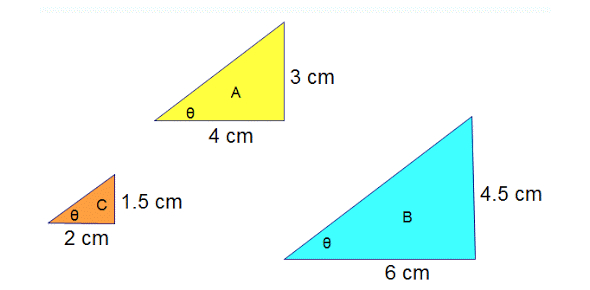# Similar And Congruent Triangles Quiz!

15 Questions | Total Attempts: 514SettingsDid you know when two objects are similar, they have the same shape but not always the same size? This similarity means we can obtain one figure from the other through a process of expansion or contraction. If the objects are the same size, they are congruent. For this quiz, you will be shown pictures of shapes, and you must answer the questions accordingly. You need to take this stellar quiz.

• 1.
Which of the following is not a criterion for congruence of triangles?
• A.

Side, Side, Side (SSS)

• B.

Angle Side Angle (ASA)

• C.

Side, Side , Angle (SSA)

• D.

Side, Angle, Side (SAS)

• 2.
In each pair of triangles, parts are congruent as marked. Which pair of triangles are congruent by ASA?
• A.

Triangle pair 1

• B.

Triangle pair 2

• C.

Triangle pair 3

• D.

Triangle pair 4

• 3.
Karabo has designed two triangular flower beds, as shown below. Which statement is true for the two flower beds?
• A.

The length of side PQ is equal to 10 feet.

• B.

They have the same perimeter.

• C.

They have different areas.

• D.

He length of side BC is equal to 10 feet.

• 4.
Among two congruent angles one has measure 70, then the measure of other angle is[Blank]
• 5.
Look at the triangle ABC. O is the center. Which statement is always correct about triangle ABC?
• A.

Segments DF and AE are congruent.

• B.

Segments AE and EC are congruent.

• C.

Segments CD and BE are congruent.

• D.

Segments BO and OE are congruent

• 6.
Two Line segments are congruent if they have the same length.
• A.

True

• B.

False

• 7.
The figure below shows the length of side DC equal to 120 units and the length of side DB equal to 160 units. What is the length of segment AC?
• A.

140 units.

• B.

180 units.

• C.

160 units.

• D.

Cannot be determined.

• 8.
In the figure shown below, segment AM bisects angle BAC, and NC is drawn parallel to AM. Which triangle is similar to Triangle BAM?
• A.

Triangle MAC.

• B.

Triangle BNC.

• C.

Triangle BAC

• D.

Triangle ACN

• 9.
When corresponding sides of two polygons are proportional, they are similar.
• A.

True

• B.

False

• 10.
Is the following triangle similar?
• A.

Yes, they are similar (SSS).

• B.

No, they're not similar.

• C.

Yes, they're similar (SAS)

• D.

Cannot be determined.

• 11.
Triangles are similar if:
• A.

All three pairs of corresponding sides are in the same proportion.

• B.

Two pairs of sides in the same proportion and the included angle equal.

• C.

They have the same shape, but cannot be different sizes.

• D.

All three pairs of corresponding angles are the same.

• 12.
The triangles are similar, solve for the question mark.
• A.

21

• B.

12.5

• C.

18

• D.

15

• 13.
A triangle has sizes measuring 11 cm, 16 cm, and 16 cm. A similar triangle has sides measuring x cm, 24 cm, and 24 cm. What is x?
• A.

X = 19 cm

• B.

X = 16.5 cm

• C.

X = 24cm

• D.

X = 16 cm

• 14.
Complete each proportion. AB / BM  =  ? / CD
• A.

MD

• B.

BM

• C.

AB

• D.

AC

• 15.
Solve for x, and y.
• A.

X = 12 y = 60

• B.

X = 145 y = 60

• C.

X = 144 y = 60

• D.

X = 144 y = 66

Related TopicsBack to top